In [ ]:


In :
import numpy as np
import matplotlib.pyplot as plt
%matplotlib nbagg


# Week 5: Periodigram and Leakage¶

We saw previously that the discrete power spectral estimate is quite imprecise, with an uncertainty of a factor of almost 40, if the data is normally distributed. This is unacceptably imprecise if you are trying to find a small-ish peak.

As with any other time when your data is too noisy, the solution is to average the data in some ways. There are two ways to do this that are commonly used. 1) is to take your raw spectrum and smooth in frequency or 2) to take a number of spectra from adjoining windows of data and average the spectra. (2) is called the periodigram, and is the focus of this lesson.

There are also issues in the finite power spectrum estimate relating to the periodicity of the data. The assumption inherent in the power spectrum is that the signal is periodic with period $T$. This is usually not the case, and if $T$ is small, the assumption can lead to artifacts in the spectrum. This is called leakage, and can be suppressed by windowing

## Frequency Smoothing¶

An obvious, and easy, method to smooth the spectrum, is to simply smooth the spectrum in frequency. We do this with a finite convolution, or a boxcar filter:

\begin{equation} \tilde{G}_{xx}\left(f_i\right) = \frac{1}{2W+1}\sum_{k=i-W}^{i+W} G_{xx}\left(f_k\right) \end{equation}

where here we have specified the boxcar to have width $2W+1$ frequency bins.

To see how this works, we create a Gaussian random noise time series and add a sine wave to it:

In :
N= 256; T = 2048.; dt = T/N  # N samples, T is time we sample for, dt is the spacing between time samples
import scipy.stats as stats
fig,ax=plt.subplots(1,1)
t=np.arange(0,T,dt)
f0=1./100.; om = 2*np.pi*f0; amp=0.5
# noise plus sine wave
x=np.random.randn(N)+amp*np.cos(om*t)
ax.plot(t,x)
ax.set_xlabel('t [s]');ax.set_ylabel('x [V]');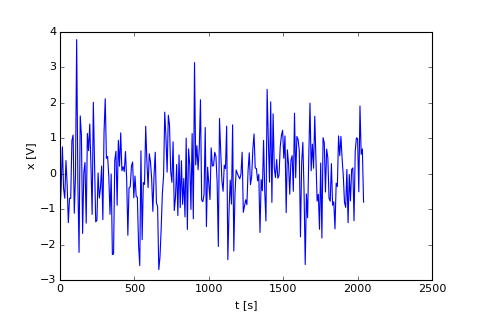Then we compute the power spectrum. Note that the theoretical spectrum for this should consist of a "white" broadband spectrum and a delta function at $f=0.01 \mathrm{Hz}$ The white part of the spectrum should integrate so that

\begin{equation} \int_{0}^{f_N} G_{xx}(f) \mathrm{d}f = 1. \end{equation}

or

\begin{equation} G_{xx}(f) = \frac{1}{f_N} \end{equation}

where $f_N=\frac{1}{2\Delta t}$ is the Nyquist frequency. The peak due to the sine wave should have a discrete amplitude given by

\begin{equation} G_{xx}(f) = \frac{a^2}{2 \delta f}\ \delta\left(f-f_0\right) \end{equation}

where $a$ is the amplitude of the sine wave, and $f_0$ is the frequency, and $\delta f=1/T$ is the spacing between discrete frequencies.

Having a theoretical spectrum is quite nice because we can check our response..

Then we calculate $G_{xx}(f)$ as described last lecture:

In :
fig,ax=plt.subplots(1,1)

f = np.arange(N/2)/T
fN = 1./2./dt
# get the theory
Gtheory = 1./fN+0.*f
ind = np.where(f>=f0)  # get the index of f0
Gtheory[ind]+=amp**2/2.*T
# get the spectral estimate:
X = dt*np.fft.fft(x)
G = (2./T)*np.real(np.conj(X[:N/2])*X[:N/2])
# plot
ax.loglog(f[1:],Gtheory[1:],color='r',label='Expected')
ax.loglog(f[1:],G[1:],label='Random')
ax.legend(loc=3)
# get the error bars:
inter = stats.chi2.interval(0.95,df=2)
ax.fill_between(f[1:],2.*G[1:]/inter,2.*G[1:]/inter,alpha=0.5,
linewidth=0.0,edgecolor=None,color=None,facecolor='b')
ax.set_xlabel('f [Hz]');ax.set_ylabel('$G_{xx}(f)$')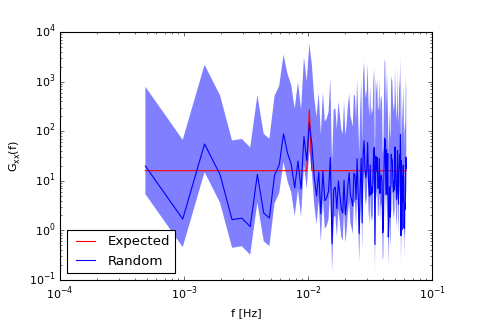Out:
<matplotlib.text.Text at 0x10cd67550>

Hopefully its clear from the above that the peak barely shows up above the variance of the spectral estimate. Certainly it is not significant with 95% confidence.

So we see if smoothing in frequency space can help improve the spectrum:

In :
W=2
W=2*W+1.
fig,ax=plt.subplots(1,1)
Gsmooth=np.convolve(G[1:],np.ones(W)/W,mode='same')
ax.loglog(f[1:],Gtheory[1:],color='r',label='Expected')
Theorysmooth=np.convolve(Gtheory[1:],np.ones(W)/W,mode='same')
ax.loglog(f[1:],Theorysmooth,'m',label='Smoothed Theory')
ax.loglog(f[1:],Gsmooth,linewidth=2,label='Freq Smooth',color='g')
inter = stats.chi2.interval(0.95,df=2*W)
ax.fill_between(f[1:],2.*W*Gsmooth/inter,2.*W*Gsmooth/inter,alpha=0.45,
linewidth=0.0,edgecolor=None,color=None,facecolor='g')
ax.legend(loc=0,fontsize='small')
ax.set_xlabel('f [Hz]');ax.set_ylabel('$G_{xx}(f)$')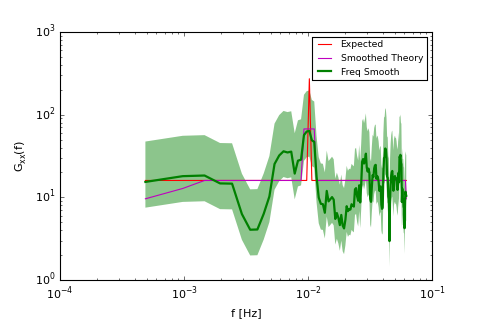Out:
<matplotlib.text.Text at 0x10d89aed0>

Here we see that the peak is above the noise. However, there is a cost to this, and that is that the peak is now wider than one frequency bin. This shouldn't be surprising because we smoothed in frequency.

Note that the lowest frequencies were not smoothed quite correctly using np.convolve. This is an edge effect of np.convolve and should probably be fixed in some intelligent manner.

Note that the error bars of the smoothed spectral estimate are narrower than the raw spectral estimate. In this case we average 5 spectral estimates, and that means we are averaging 10 squared Normal distributions:

$$\tilde{G}_{xx}(f_k) = \frac{1}{5}\left(G_{xx}(f_{k-2})+G_{xx}(f_{k-1})+G_{xx}(f_{k})+G_{xx}(f_{k+1})+G_{xx}(f_{k+2})\right)$$

so this is distributed as a $\chi^2_{10}$ random variable, from which we get the error bounds pltted in green.

So, there is an inherent tradeoff - less variance in the spectral estimate comes at the cost of poorer frequency resolution.

## Block Averaging (Welch's Method)¶

Another method of decreasing the variance of the spectral estimate is to split the data up into "blocks", compute spectral estimates, and then average the spectral estimates from the blocks to create an estimate with less spectral variance. Doing this is relatively straight forward. Note here we make a longer time series, and make $f_0$ of our synthetic signal higher:

In :
N= 2560
T = 2048.
dt = T/N

fig,ax=plt.subplots(1,1)
t=np.arange(0,T,dt)
f0=1./30.;    om = 2*np.pi*f0;    amp=0.5
x=np.random.randn(N)+amp*np.cos(om*t)
ax.plot(t,x,'k')
NFFT=256
# there is a better way to do this, but lets brute force it:
nblock = np.floor(N/NFFT)
print nblock
for ind in range(int(nblock)):
inds =range(ind*NFFT,(ind+1)*NFFT)
tblock=t[inds]
xblock=x[inds]
ax.plot(tblock,xblock)
ax.set_xlabel('t [s]');ax.set_ylabel('X [V]')
plt.axis('tight')
fig.tight_layout()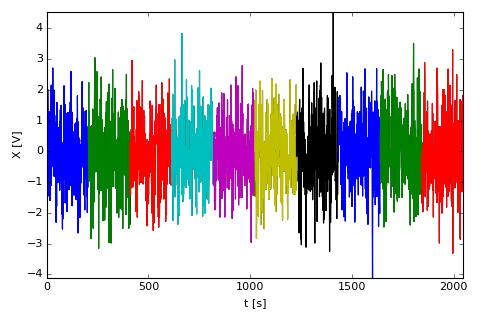10.0


Here we have coloured our 10 blocks of data that we will take independent periodigrams of. (Note that we cannot average the data and take the periodigram and expect to get anything sensible).

Then we compute the power spectra for the 10 blocks and plot. We will also plot the raw periodigram:

In :
Gblock=np.zeros((nblock,NFFT/2))
fig,ax=plt.subplots(1,1)

# raw:
X = dt*np.fft.fft(x)
Graw = (2./T)*np.real(np.conj(X[:N/2])*X[:N/2])
f=np.arange(N/2)/T

ax.loglog(f,Graw,'0.5',linewidth=2)
# blocks:
for ind in range(int(nblock)):
inds =range(ind*NFFT,(ind+1)*NFFT)
tblock=t[inds]
xblock=x[inds]
X = dt*np.fft.fft(xblock)
Tblock=T/nblock
Gblock[ind,:] = (2./Tblock)*np.real(np.conj(X[:NFFT/2])*X[:NFFT/2])
fblock= np.arange(NFFT/2)/Tblock
ax.loglog(fblock[1:],Gblock[ind,1:])
ax.set_xlabel('f [Hz]');ax.set_ylabel('$G_{xx}(f)\ \ [V^2\,Hz^{-1}$')

/Users/jklymak/anaconda/lib/python2.7/site-packages/ipykernel/__main__.py:1: DeprecationWarning: using a non-integer number instead of an integer will result in an error in the future
if __name__ == '__main__':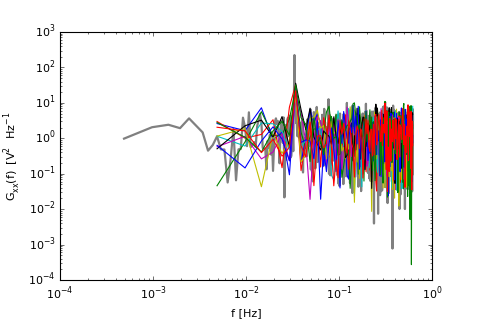Out:
<matplotlib.text.Text at 0x10e958f50>

By eye, it is not clear that we have improved our spectral estimate. The individual blocks yield noisy spectra estimates. In fact it is easy to show they are just as noisy as the full spectrum.

However, taking the average yields a much smoother spectrum. Note the degrees of freedom is $2N_{blocks}$.

In :
# make the theory spectrum:
fN=1/2./dt
Gtheory = 1./fN+0.*f
ind = np.where(f>=f0)
Gtheory[ind]+=amp**2/2.*T

fig,ax=plt.subplots(1,1)
ax.loglog(f,Graw,color='0.5')
ax.loglog(f,Gtheory,'r')
# get error bars:
dof=2*nblock
inter = stats.chi2.interval(0.95,df=dof)
Gblockm=np.mean(Gblock,axis=0)
ax.fill_between(fblock[1:],dof*Gblockm[1:]/inter,dof*Gblockm[1:]/inter,alpha=0.45,
linewidth=0.0,edgecolor=None,color=None,facecolor='g',zorder=3)
ax.loglog(fblock,Gblockm,'k',linewidth=2,zorder=10)
ax.set_xlabel('f [Hz]');ax.set_ylabel('$G_{xx}(f)\ \ [V^2\,Hz^{-1}$')
ax.set_title('%d blocks = %d degrees of freedom'%(nblock,2*nblock))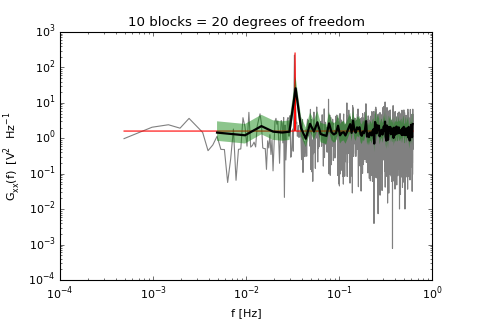Out:
<matplotlib.text.Text at 0x10fd83b10>

So, some things to note:

1. The block-spectra do not extend to as low frequencies as the raw periodigram. This should not be surprising because the raw periodigram is taking a longer data set than the individual blocks. Indeed $f_1=\delta f = 1/T$, so the longer $T$ the lower a frequency can be resolved.
2. The resolution of the bloc spectra is also less than the raw. i.e. we get the same tradeoff as smoothing in frequency - we lose frequency resolution, again because $\delta f = 1/T$.

So, is this better than frequency smoothing? The question depends on what you are trying to do and what your signal is like. If you need the low frequency information, then the block-average method is obviously problematic. However, as we saw above, you also need to be careful how you "average" those lower few bins. Do they give the same result?

In :
fig,ax=plt.subplots(1,1)
ax.loglog(f,Graw,color='0.5')
ax.loglog(f,Gtheory,'r')
# get error bars:
dof=2*nblock
inter = stats.chi2.interval(0.95,df=dof)
Gblockm=np.mean(Gblock,axis=0)
ax.fill_between(fblock[1:],dof*Gblockm[1:]/inter,dof*Gblockm[1:]/inter,alpha=0.45,
linewidth=0.0,edgecolor=None,color=None,facecolor='g',zorder=3)
ax.loglog(fblock,Gblockm,'k',linewidth=2,zorder=2)
ax.set_xlabel('f [Hz]');ax.set_ylabel('$G_{xx}(f)\ \ [V^2\,Hz^{-1}$')
ax.set_title('%d blocks = %d degrees of freedom'%(nblock,2*nblock))
Gsmooth = np.convolve(Graw,np.ones(10)/10.,mode='same')
ax.loglog(f,Gsmooth,'b',label='Smoothed',zorder=4)
ax.loglog(f[::10],Gsmooth[::10],'m',label='Smooth decimated',zorder=5,linewidth=3)
ax.set_xlim([1e-2,1e-1])
ax.legend(loc=3)
fig.show()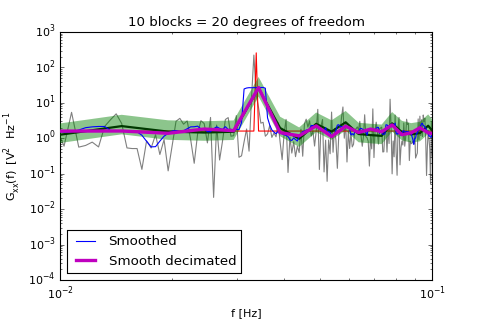There is no statistical difference between smoothing and block-averaging, and one could argue that the frequency resolution of smoothing is somewhat superior to block averaging because the wide smoothed "peak" will always be centered on the data peak.

So why do the block averaging? The reason is probably historical, but it also has to do with spectral bias or leakage.

## Leakage and Bias of the periodigram¶

There is a big problem with the periodigram, and it is that we are approximating a finite time series as periodic functions. What this means is that we are eseentially assuming that after the end of our time series, it repeats all over again. Consider a time series with a lot of low-frequency information - it will have a sharp discontinuity at $t=T$:

In :
N= 128; T = 2048.; dt = T/N
np.random.seed(seed=456)
x=np.cumsum(np.random.randn(N)*dt)
t = np.arange(0,T,dt)
tt=[]; xx=[]
for ind in range(4):
tt=np.hstack((tt,t+ind*T))
xx=np.hstack((xx,x))
fig,ax=plt.subplots(1,1)
ax.plot(tt,xx,'k')
for ind in range(4):
ax.plot(t+ind*T,x);
ax.set_xlabel('t [s]');ax.set_ylabel('x [V]')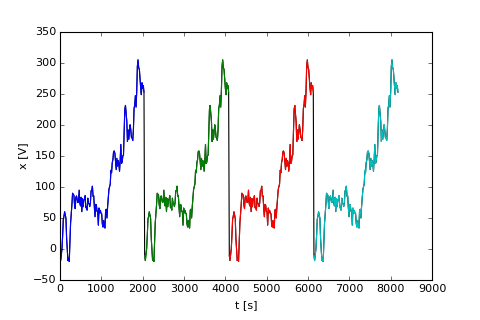Out:
<matplotlib.text.Text at 0x114526790>

There is a large discontibuity at $t=T=2048 s$. This discontibuity shows up in the spectra. Similarly if we consider a sine wave:

In :
N= 2049;T = 2048.;dt = T/N;om = 2*np.pi/600.
t = np.arange(0,T,dt);x=np.sin(om*t)
tt=[];xx=[]
for ind in range(3):
tt=np.hstack((tt,t+ind*T))
xx=np.hstack((xx,x))
fig,ax=plt.subplots(1,1)
ax.plot(tt,xx,'k');ax.plot(t,x,'r');ax.plot(t+T,x,'b');ax.plot(t+T*2,x,'g')
ax.set_xlabel('t [s]');ax.set_ylabel('x [V]')

Out:
<matplotlib.text.Text at 0x1096b1090>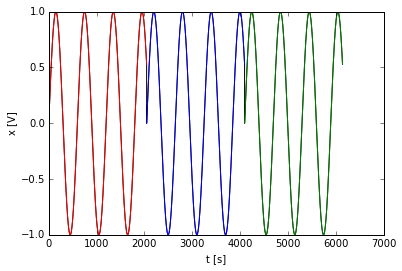Again the discontiuty at $t=T$ is going to show up in our spectral estimation.

For the single frequency, lets consider the raw periodigram of the signal.

NOTE: below we will define a function to do the raw periodigram steps outlined above.

In :
def rawperio(x,fs):
dt=1./fs
N = len(x)
T = N*dt
X = dt*np.fft.fft(x)
G = (2./T)*np.real(np.conj(X[:N/2])*X[:N/2])
f= np.arange(N/2)/T
return G[1:],f[1:]

In :
N= 2049;T = 2048.;dt = T/N;
f=np.arange(N/2)/T
df=1./T
f0=f+df/3.
om = 2*np.pi*(f0)
t = np.arange(0,T,dt)
x=np.sin(om*t)
G,f=rawperio(x,1./dt)
fig,ax=plt.subplots(1,1)
ax.loglog(f,G,'k.',markersize=10)
ax.loglog(f,G,'k')
ax.plot(np.array([1,1])*f0,[1e-4,1e4],'r')
ax.set_ylim([1e-3,1000.]);ax.set_xlim([min(f),0.1])
ax.set_xlabel('f [Hz]');ax.set_ylabel('$G_{xx} [V^2 Hz^{-1}]$')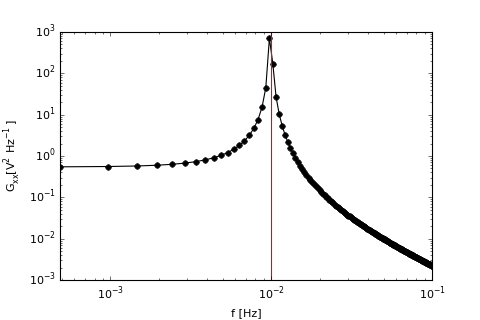Out:
<matplotlib.text.Text at 0x114d31a50>

The peak is well-defined, but there is substantial energy at frequencies that are off the peak. These roll off, but in some applications this roll-off may not be fast enough. This is what is meant by "spectral leakage".

### Leakage derived analytically¶

We can derive the effect of a finite sample of length $T$ analytically if we think about it as a window from $t=0$ to $T=1$ on an infinite time series. So, suppose we have a signal $x(t)$ that exists for all $t$, then the periodigram just looks at a shorter signal $x_T(t)$:

\begin{equation} x_T(t) = u_T(t)x(t) \end{equation}

where $u_T(t)=1$ when $0\leq t\leq T$, and $u_T(t)=0$ otherwise.

Taking the Forurier transform and using the convolution theorem yields

\begin{equation} X_T(f) = U_T(f)\star X(f) \end{equation}

or that the Fourier transform is the true Fourier transform convolved with the Fourier transform of the window. $U_T(f)$ is easy to compute:

\begin{align} U_T(f)&= \int_{-\infty}^{\infty} \mathrm{e}^{-j2\pi f t} \mathrm{d}t\\ &= \int_0^T \mathrm{e}^{-j2\pi f t} \mathrm{d}t\\ &= T\mathrm{e}^{-j\pi fT} \int_{-1/2}^{1/2} \mathrm{e}^{-j2\pi fT t'} \mathrm{d}t' \\ &= T\mathrm{e}^{-j\pi fT} \frac{\mathrm{sin}(\pi fT)}{\pi fT} \end{align}

where we said that $t'=t/T-1/2$. This ratio comes up a lot, and therefore we write:

\begin{align} U_T(f)&= T\mathrm{e}^{-j\pi fT} \mathrm{sinc}\left( fT\right) \end{align}

So what happens for our sine wave above? This has a Fourier transform of $X(f)=\delta(f-f_0)$, so the Fourier transform is $X_T(f)=T\mathrm{e}^{-j\pi (f-f_0) T} \mathrm{sinc}\left( (f-f_0)T\right)$, or the power spectral density is

\begin{equation} S_{xx}(f,T)=\frac{T}{2}\ \mathrm{sinc}^2\left((f-f_0)T\right) \end{equation}

Note that

\begin{equation} \int_{-\infty}^{\infty} S_{xx}\ \mathrm{d}f = \frac{1}{2} \end{equation}

which is the variance of a sine wave. The sinc function has zeros at $f-f_0=nT$ where $n$ is any integer not equal to zero.

NOTE: different people define "sinc" differently, usually with or without the $\pi$ as an argument. Numpy's np.sinc uses the definition here:

\begin{equation} \mathrm{sinc}(x)=\frac{\sin(\pi x)}{\pi x} \end{equation}
In :
f0=1.
fig,ax=plt.subplots(1,1)
f=np.linspace(-20.,20.,100000)
for T in [2,5,10,20]:
thesinc=T*np.sinc((f-f0)*T)**2/2.
df = np.median(np.diff(f))
ax.plot(f,thesinc,label='$T=%d [s]$'%T)
ax.set_xlim([0,2]);ax.set_ylim([0,11]);ax.legend()
ax.set_xlabel('$f\ [Hz]$');ax.set_ylabel('$S_{xx}(f)\ \ [V^2 Hz^{-1}]$')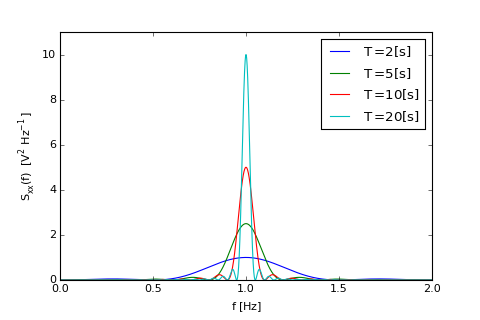Out:
<matplotlib.text.Text at 0x115a5d090>

This is the effect of calculating the power spectrum of a finite time series. There is nothing about discretizing the time series in here yet. Note that as $T$ gets larger, the power spectrum more closely approaches a delta function, with the "sidelobes" closer to fundamental peak, and the fundamental peak gets much taller. The fundamental peak is $T/2$ high.

The "sidelobes" are the subsidiary peaks on either side of the main peak. These sidelobes are highest at when $\left|\sin(\pi (f-f_0)T)\right|\approx 1$, or when $f-f_0\approx\frac{2n+1}{2T}$, except for the first time, which is burried in the roll off, so $\left|n\right|>0$. The sidelobe amplitudes are approximately

\begin{equation} 2T\left(\frac{1 }{\pi (2n+1)}\right)^2 \end{equation}

or $4/\left(\pi (2n+1)\right)^2$ of the peak height. So the first sidelobe, $n=1$ is $0.045$ of the peak value, or $10\mathrm{log}_{10}(0.045)=-13.5$ dB. The second side lobe is at $n=2$, or $f-f_0 \approx \frac{5}{2T}$ and is $0.016$ of the peak or -18 dB.

These sidelobes explain the energy that leaks into the frequencies around the peak as shown below:

In :
N= 2049;T = 2048.;dt = T/N;
fig,axs=plt.subplots(1,2)

for ind,ax in enumerate(axs):
f=np.arange(N/2)/T
df=1./T
if ind==0:
om = 2*np.pi*(f+0.3*df)
else:
om = 2*np.pi*(f)
t = np.arange(0,T,dt);    x=np.sin(om*t)
G,f=rawperio(x,1./dt)
ax.loglog(f,G,'db')
ax.step(f,G,where='mid');
f0=om/2./np.pi
ff=np.logspace(-4,1,10000)
thesinc=T*np.sinc((ff-f0)*T)**2/2.
ax.loglog(ff,thesinc);
localsinc=np.interp(f,ff,thesinc)
ax.loglog(f,localsinc,'d')
ax.set_xlim([8e-3,1.3e-2]);ax.set_ylim([1.e-1,1e4]);    ax.set_title('$f_0= %1.2f\ \delta f$'%(f0/df))
ax.set_xlabel('f [Hz]');ax.set_ylabel('$G_xx(f)$')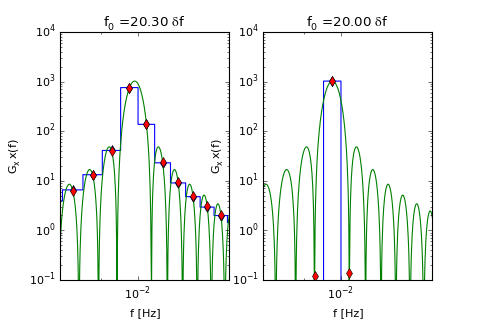Here we see that the discrete periodigram we calculate is projected onto the sinc function at the frequencies $f_k = k\delta f$. Usually this leads to off-peak frequencies having spectral energy. A special case occurs when the frequency of the sine wave $f_0$ is an integer multiple of the periodigram frequency spacing $\delta f$. In that case, shown above on the right, the periodigram just has one peak because the off-peak entries are at the nulls in the periodigram.

In the case on the left, we might not mind the central two frequency bins splitting the energy of the sine wave. After all, the true frequency lies between them. However, we might mind the lobes to the left and the right, as they may obscure other peaks or a background continuum spectrum.

In the example below, our spectrum consists of two peaks and some background noise. The peak at the lower frequency is considerably smaller.

In :
t = np.arange(0,T,dt);
om1 = 2*np.pi*(f+0.3*df)
om2 = 2*np.pi*(f+0.3*df)
x=np.sin(om1*t)+0.005*np.sin(om2*t+0.1)+np.random.randn(len(t))/100.
G,f=rawperio(x,1./dt)
xback=0.*np.sin(om1*t)+0.005*np.sin(om2*t+0.1)+np.random.randn(len(t))/100.
Gg,f=rawperio(xback,1./dt)
df=np.median(np.diff(f))
fig,ax=plt.subplots(1,1)
ax.loglog(f,Gg,label='Periodigram w/o main peak')
ax.loglog(f,G,label='Periodigam')
ax.set_xlabel('f [Hz]');ax.set_ylabel('$G_xx(f)$')
ff = np.logspace(np.log10(f),np.log10(f[-1]),10000)
theory = 0*ff+1./100.**2/f[-1]
ind = np.where(ff>=om1/2./np.pi)
theory[ind]=0.5/df
ind = np.where(ff>=om2/2./np.pi)
theory[ind]=0.5/df*0.015**2
ax.loglog(ff,theory,label='Theory')
ax.legend(loc=2,fontsize='small')
ax.set_xlabel('f [Hz]');ax.set_ylabel('$G_xx(f)$');ax.set_ylim([1e-4,0.5e4])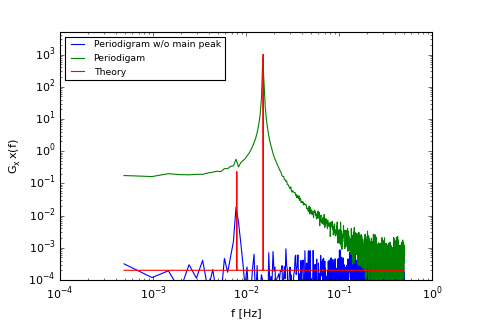Out:
(0.0001, 5000.0)

The weaker peak is definitely well above the "noise" of our process, but is obscured by the sidelobe leakage from the dominant peak in the green curve.

The solution to this problem is to smooth out the discontibuities at the edge of the spectrum by applying a window that makes the edge of the window go to zero.

## Windows to reduce sidelobe leakage¶

So the solution is to apply a window that reduces the sidelobe leakage. Here we apply the Hanning window:

\begin{equation} w(n)=0.5\left(1-\cos \left(\frac{2\pi n}{N-1} \right) \right) \end{equation}

where $n=0,1...N-1$, and $N$ is the length of the time series. Before describing it, lets see the effect on the powerspectrum calculated above:

In :
fig,ax=plt.subplots(1,1)
ax.loglog(f,Gg,label='Periodigram w/o main peak'); ax.loglog(f,G,label='Periodigam'); ax.set_xlabel('f [Hz]');ax.set_ylabel('$G_xx(f)$')
ff = np.logspace(np.log10(f),np.log10(f[-1]),10000)
ax.loglog(ff,theory,label='Theory')
ax.legend(loc=2,fontsize='small')
ax.set_xlabel('f [Hz]');ax.set_ylabel('$G_xx(f)$');ax.set_ylim([1e-4,0.5e4])
han0 = 0.5*(1-np.cos(2*np.pi*np.arange(0,N)/(N-1)))
han = han0/np.sqrt(np.sum(han0**2/N))
Gg,f=rawperio(x*han,1./dt)
ax.loglog(f,Gg,'c',linewidth=2,label='Hanning')
ax.legend(loc=2,fontsize='small')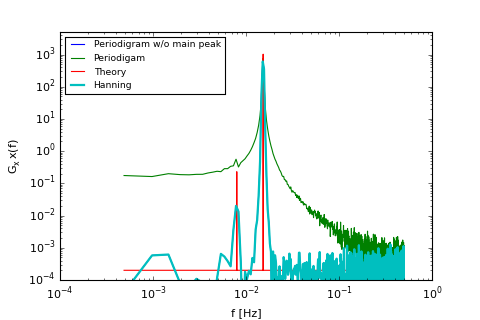Out:
<matplotlib.legend.Legend at 0x11866aa90>

We see that the sidelobe leakage is greatly reduced, and the smalled peak is clearly discernable. Furthermore, the background noise level (the flat line) is clear in this spectrum as well.

The raw periodigram is basically applying a boxcar window to the infinite time series, and the windowed periodigram is applying a more tapered window. Note that the Hanning window tapers to zero at the ends. This gets rid of the discontinuities in the implied time series:

In :
def plotit():
# convenience so we can replot below
T=1.;N=2048;dt=T/N;f0=0
fig,axs=plt.subplots(1,2)
ax=axs
tt=np.arange(-0.5*T,1.5*T,dt);xx = 0*tt;xx[(tt>=0)&(tt<=T)]=1.
ax.plot(tt,xx)
wind = 0.5*(1-np.cos(2*np.pi*np.arange(0,N)/(N-1)));wind = wind/np.sqrt(np.sum(wind**2/N))
xx[(tt>=0)&(tt<T)]=wind
ax.plot(tt,xx)
ax.set_xlabel('t [s]');ax.set_ylabel('w[t]');ax.axis('tight')

ax=axs
f=np.linspace(-3./T,6./T,100000)
thesinc=T*np.sinc((f-f0)*T)**2/2.
ax.semilogy(f,thesinc,label='Boxcar')
ax.legend(loc=1,fontsize='small')
ax.set_ylim([1e-0,1.5e3]);ax.set_ylabel('$W(f)^2$');ax.axis('tight');ax.set_ylim([1e-5,1.2]);ax.set_xlabel('f T');plt.tight_layout()
return fig,ax
fig,ax=plotit()